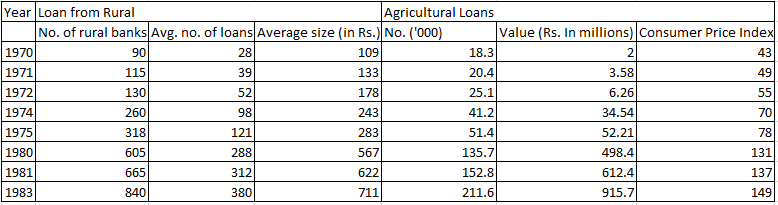### CAT 1997 Question Paper Question 30

Instructions

The table given below gives the annual details of loans from rural banks and agricultural loans over the years 1970 to 1983. Using this data answer the questions that follow.Question 30

# If the consumer price index for 1970 is to be taken as 105 and the indices for the subsequent years are to be corrected accordingly, what is the approximate value of the agricultural loans in 1980 at 1983 prices?

Solution

Since the consumer price indices for all years change, the ratios will remain the same as before.

Value of the agricultural loans in 1980 at 1983 prices = 498.4 * 149 / 131 = 570 approx.

• All Quant CAT Formulas and shortcuts PDF
• 30+ CAT previous papers with solutions PDF